Special Right Triangle Practice Terms of Use    Contact Person: Donna Roberts1.
Given the diagram at the right, as labeled, find x and y.

Choose:
 x =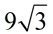;   y = 9 x = 9;    y =x =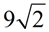;    y = 9 x = 9;    y =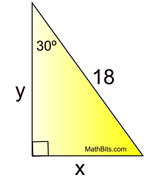2.
Given the diagram, as labeled, at the right.
Find x.

Choose:
 4 4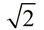8 8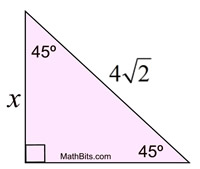3.
Given square ABCD with a side length of 10 inches. Find the diagonal length AC.

Choose:
 510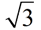510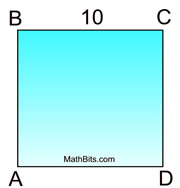4.
Rhombus ABCD has mABC = 120º and
AB = 7. Find diagonal length, BD.

Choose:
 7 79 9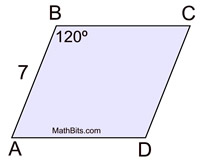5.
Given trapezoid ABCD, with
m
A = 45º, AB = 16 inches. and
BC = 18 inches.
Find the area of the trapezoid to the
nearest square inch .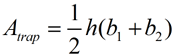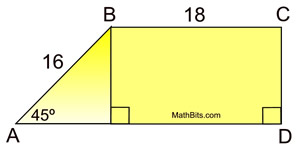Choose:
 144 sq.in. 176 sq.in. 268 sq.in. 352 sq.in.

6.
The altitude of an equilateral triangle is 9 inches long. Find the perimeter of the triangle.

Choose: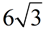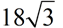27 54

7.
In the diagram below, points A, B, C and D are collinear.
Find x and y.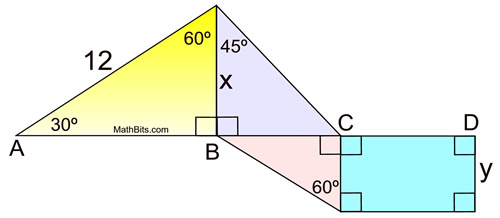Choose:
 x = 6 and y = 6 x = 6 and y = 3 x = 6 and y =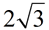x = 6 and y =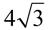8.
Martin walks his dog on level ground in a straight line with the dog's favorite tree. The angle of elevation from Martin's present location to the top of a nearby telephone pole is 30º. The angle of elevation from the base of the tree to the top of the telephone pole is 45º. If the telephone pole is 42 feet tall, how far are Martin and his dog from the tree. Express the exact length.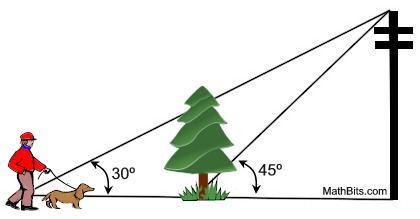Choose:
 84 424242- 42

9.
Given the diagram at the right as labeled.
Find x.

Choose:
 36 66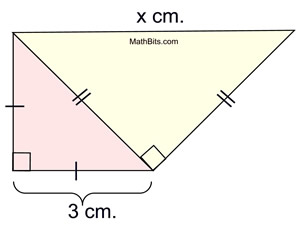10.
Given the diagram at the right.
a) Find a.
Choose:
 16 88 16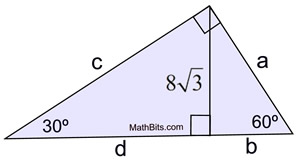b) Find b.
Choose:
 16 8 168c)
Find c.
Choose:
 16 24 168d)
Find d.
Choose:
 16 24 168